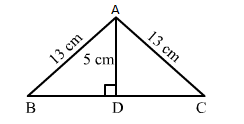# ∆ABC is an isosceles triangle with AB = AC = 13 cm and the length of altitude fromQuestion:

ABC is an isosceles triangle with AB = AC = 13 cm and the length of altitude from A on BC is 5 cm. Then, BC = ?
(a) 12 cm
(b) 16 cm
(c) 18 cm
(d) 24 cm

Solution:

(d) 24 cmIn triangle ABC, let the altitude from A on BC meets BC at D.
We have:
AD = 5 cm, AB = 13 cm and D is the midpoint of BC.
Applying Pythagoras theorem in right-angled triangle ABD, we get:

$A B^{2}=A D^{2}+B D^{2}$

$\Rightarrow B D^{2}=A B^{2}-A D^{2}$

$\Rightarrow B D^{2}=13^{2}-5^{2}$

$\Rightarrow B D^{2}=169-25$

$\Rightarrow B D^{2}=144$

$\Rightarrow B D=\sqrt{144}=12 \mathrm{~cm}$

Therefore, BC = 2BD = 24 cm